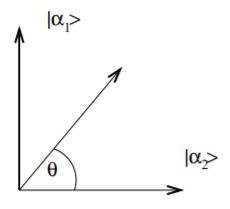# 3.5: Time-variation of expectation values - Degeneracy and constants of motion

$$\newcommand{\vecs}{\overset { \rightharpoonup} {\mathbf{#1}} }$$ $$\newcommand{\vecd}{\overset{-\!-\!\rightharpoonup}{\vphantom{a}\smash {#1}}}$$$$\newcommand{\id}{\mathrm{id}}$$ $$\newcommand{\Span}{\mathrm{span}}$$ $$\newcommand{\kernel}{\mathrm{null}\,}$$ $$\newcommand{\range}{\mathrm{range}\,}$$ $$\newcommand{\RealPart}{\mathrm{Re}}$$ $$\newcommand{\ImaginaryPart}{\mathrm{Im}}$$ $$\newcommand{\Argument}{\mathrm{Arg}}$$ $$\newcommand{\norm}{\| #1 \|}$$ $$\newcommand{\inner}{\langle #1, #2 \rangle}$$ $$\newcommand{\Span}{\mathrm{span}}$$ $$\newcommand{\id}{\mathrm{id}}$$ $$\newcommand{\Span}{\mathrm{span}}$$ $$\newcommand{\kernel}{\mathrm{null}\,}$$ $$\newcommand{\range}{\mathrm{range}\,}$$ $$\newcommand{\RealPart}{\mathrm{Re}}$$ $$\newcommand{\ImaginaryPart}{\mathrm{Im}}$$ $$\newcommand{\Argument}{\mathrm{Arg}}$$ $$\newcommand{\norm}{\| #1 \|}$$ $$\newcommand{\inner}{\langle #1, #2 \rangle}$$ $$\newcommand{\Span}{\mathrm{span}}$$$$\newcommand{\AA}{\unicode[.8,0]{x212B}}$$

The time variation of the expectation value of an operator $$\hat{A}$$ which commutes with the Hamiltonian is:

$\frac{d}{dt} \langle \Phi |\hat{A}| \Phi \rangle = \int d^3 r \frac{d \Phi^*}{dt} \hat{A} \Phi + \Phi^*\hat{A} \frac{d \Phi}{dt} \nonumber$

but since $$i \hbar \frac{d \Phi}{dt} = \hat{H} \Phi$$ and $$−i \hbar \frac{d \Phi^*}{dt} = \hat{H}^* \Phi^*$$

$−i \hbar \frac{d}{dt} \langle \Phi |\hat{A}| \Phi \rangle = \int (\hat{H}^* \Phi^*\hat{A} \Phi − \Phi^* \hat{A}\hat{H} \Phi )d^3 r = h \Phi |[\hat{H}, \hat{A}]| \Phi \rangle \nonumber$

Where we also use the fact that $$\hat{H}$$ is Hermitian. Thus if $$\hat{H}$$ commutes with $$\hat{A} ([\hat{H}, \hat{A}] = 0)$$, the expectation value of A is independent of time. It is a conserved quantity.

As we have seen above, if we have degenerate eigenstates of the Hamiltonian, $$\hat{H}$$, then there must be some other operator $$\hat{A}$$ which commutes with the Hamiltonian for which there are energydegenerate eigenstates with different eigenvalues A. These eigenvalues, A, are then constants of the motion. Moreover, if $$\Phi$$ is an eigenfunction of $$\hat{H}$$, then $$\hat{A} \Phi$$ is also an eigenfunction of $$\hat{H}$$.

$\hat{H} (\hat{A} \Phi ) = \hat{A}\hat{H} \Phi = \hat{A}(E \Phi ) = E(\hat{A} \Phi ) \nonumber$

There is a three way link between symmetry, degeneracy and conserved quantities.Figure $$\PageIndex{1}$$: Any linear combination of two degenerate eigenstates produces another eigenstate.

This page titled 3.5: Time-variation of expectation values - Degeneracy and constants of motion is shared under a CC BY 4.0 license and was authored, remixed, and/or curated by Graeme Ackland via source content that was edited to the style and standards of the LibreTexts platform; a detailed edit history is available upon request.